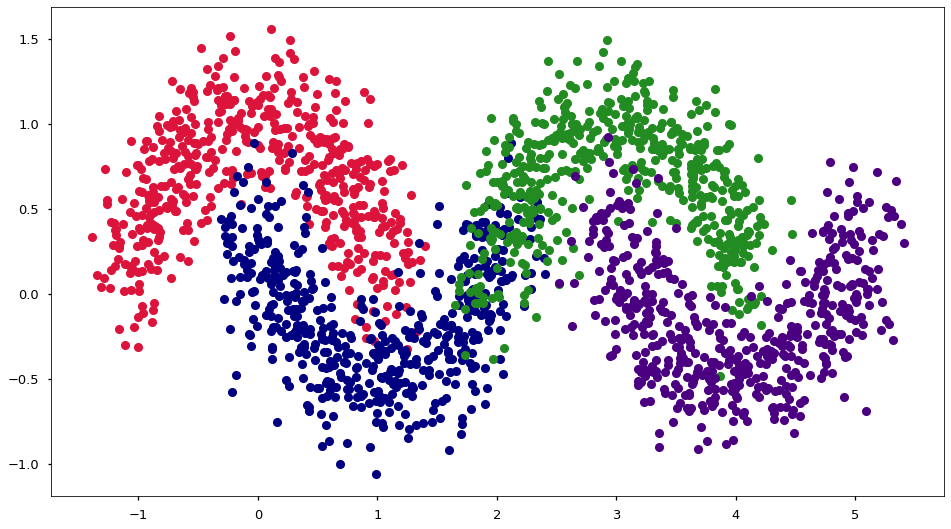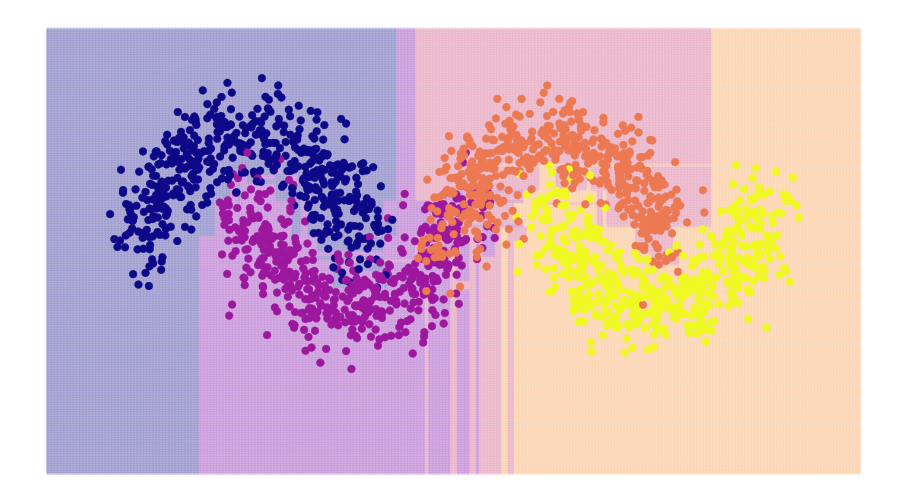# 09.01 Trees¶

Before we attempt to describe how one uses several models at once to solve a single problem we need to take a step back and look at a rather simple but still useful model, the decision tree.sl-major-oak.svg
The Royal Oak is a major Oak tree in Sherwood Forest. Legend says that Robin Hood and his band of Merry Men had their hideout below the tree. The Oak itself is at least 800 years old which makes it viable for it to be alive and reasonably grown during the time the legendary folk hero supposedly lived. Whether Robin Hood himself lived or whether his tales are a collection of stories aggregated from the deeds of many people remains unsolved. One thing we can say rather confidently about Robin Hood is that he very likely did wear green clothes. In the 13th century hunters in the forests in the Midlands used clothes dyed in Lincoln, which was famous for green dyes.

For a start we import the usual stuff. We will again use the moons dataset creator and take the decision tree classifier to work on a non-linear problem.

In :
import numpy as np
import matplotlib.pyplot as plt
%matplotlib inline
plt.style.use('seaborn-talk')
from sklearn.datasets import make_moons
from sklearn.tree import DecisionTreeClassifier
from sklearn.model_selection import cross_val_score, KFold


Two moons are a non-linear problem but we can produce a more complex problem by merging two two moons datasets into a four moon dataset. The decision tree is a model which can separate several classes at once, and with four moons we have four distinct classes.

Note that we moved the second set of moons to the right by adding $3$ to it. And that we also add to the labels of the second set of moons in order to have four different classes.

In :
def make_four_moons(n, noise):
X_left, y_left = make_moons(n//2, noise=noise)
X_right, y_right = make_moons(n//2, noise=noise)
X_right[:, 0] += 3
y_right[:] += 2
X = np.vstack((X_left, X_right))
y = np.hstack((y_left, y_right))
return X, y


Let's have a look how this turns out.

In :
X, y = make_four_moons(2048, noise=.2)
fig, ax = plt.subplots(figsize=(16, 9))
colors = ['crimson', 'navy', 'forestgreen', 'indigo']
for i in range(4):
ax.plot(X[y == i, 0], X[y == i, 1], 'o', c=colors[i])A decision tree is a rather simple non-parametric model, either used as a classifier or a regressor. During training a decision tree learns rules that divide the feature space perpendicular to a feature. In other words, at each training step, the tree divides one dimension into two groups.

At the first training step the tree divides the entire feature space. On all following steps the tree attempt to divide each resulting divided branch further. This happens until all leaves contain only data with the same label, or another stopping criteria is reached. A handful of stopping criteria can be the depth (or height) of the tree, which limits how many times the tree can split; the minimum number of data points in a leaf, which is the smallest possible split produced by a tree.

There are several algorithms to train a decision tree. The algorithms are concerned in how, at each step, perform the best split. At each step a tree can split the feature space into two separate areas using any of the features. Therefore each feature is evaluated for the best split so that as many labels are separated, then an information gain measure is used to decide which split produces the tree that better slits classes apart. The information gain measure differs between algorithms, for classification we have a handful of information measures.

Take the split which minimizes the gini impurity,

$$\sum_k \left[ \frac{\sum_i (y_i = k)}{N} \left( 1 - \frac{\sum_i (y_i = k)}{N} \right) \right]$$

or, take the split which minimizes the entropy

$$\sum_k \left[ - \frac{\sum_i (y_i = k)}{N} log \left( \frac{\sum_i (y_i = k)}{N} \right) \right]$$

or even the split which minimizes the maximum misclassification error.

$$\sum_k \left[ 1 - max \left( \frac{\sum_i (y_i = k)}{N} \right) \right]$$

Where $k$ is each class and $N$ the number of samples being split. For regression criteria such as mean square error can be used.

Once a tree performs a split then the division is set in stone. Each side of the split is then evaluated for more splits taking into account only the data points that are inside that split. In other words, once a tree has split the dataset into two parts (splits) each part (split) is considered a new complete dataset and a new decision tree split is performed on it as if it were a new tree. The final complete tree is a connection of all these splits into a branched structure.

No matter the split method the algorithm is always a greedy algorithm. The tree will always attempt the split that will reduce the impurity/entropy the most at the current step. By no means this guarantees that the final tree will be optimal, or have the minimum overall impurity/entropy.

In general decision trees tend to overfit the data. The stopping criteria are ways of making the tree not overfit the data but the best criteria can be hard to find, i.e. by hyperparamter search. Without any criteria we will just split the data as much as we can.

We have the data and we know something about decisions trees. Other than the internals of the model, in sklearn trees work with the same fit and predict methods.

In :
model = DecisionTreeClassifier()
cross_val_score(model, X, y, cv=KFold(n_splits=5, shuffle=True))

Out:
array([0.90731707, 0.91707317, 0.9195122 , 0.91198044, 0.9193154 ])

Good enough for such a simple classifier. As most sklearn classes the decision tree classifier has some sensible defaults.

Decision trees are simple and computationally cheap to use for multilabel classification, also this is a model that can estimate class probabilities. We can also extract the rules the tree has learned to explain what it is doing. In other words, the decision tree is a white-box model, a model that can explain how it performs the classification.

We can look at how the classification happened. In the plot we use color maps in two ways. In one we assign cmap= and the engine figures out that it needs to split the color in four different hues. Another way to do it is to take the color map from the cm module and execute it with color an array of values between $0$ and $1$. Also, there are more efficient ways to plot an entire canvas of color, with contour and filled contours procedures from matplotlib. But when it doubt the trick of plotting many semi-transparent points - as we do here - make it easier to spot visualization mistakes.

In :
model = DecisionTreeClassifier()
model.fit(X, y)

fig, ax = plt.subplots(figsize=(16, 9))
gx = np.linspace(-2, 6, 256)
gy = np.linspace(-2, 2, 256)
gx, gy = np.meshgrid(gx, gy)
gx, gy = gx.reshape(-1), gy.reshape(-1)
z = model.predict(np.c_[gx, gy])
ax.scatter(gx, gy, c=plt.cm.plasma(z / 4), alpha=0.2, s=10)
ax.scatter(X[:, 0], X[:, 1], s=60, c=y, cmap='plasma')
ax.axis('off');And we can see a lot of overfitting in the middle of the figure. Since the data is two dimensional we can visualize it and argue for specific stopping conditions. I here a good choice would be to not allow leaves with less than, say, $10$ data points.

Unfortunately, as we did see, visualizing a dataset can be quite hard. Soon we will see how to avoid the a good deal of the overfitting without the need for visualization. For the time being let's look at how the decision tree decided to split the data. The max_depth= hyperparamter is a stopping criteria, and we will use it to build our tree by parts.

In :
fig, ax = plt.subplots(2, 3, figsize=(16, 9))
gx = np.linspace(-2, 6, 256)
gy = np.linspace(-2, 2, 256)
gx, gy = np.meshgrid(gx, gy)
gx, gy = gx.reshape(-1), gy.reshape(-1)

for i in range(6):
model = DecisionTreeClassifier(max_depth=i+1)
model.fit(X, y)
z = model.predict(np.c_[gx, gy])
ax.flat[i].scatter(gx, gy, c=plt.cm.plasma(z / 4), alpha=0.1, s=10)
ax.flat[i].scatter(X[:, 0], X[:, 1], s=10, c=y, cmap='plasma')
ax.flat[i].set_title('split %s' % (i+1))
ax.flat[i].axis('off')